International Journal of Intelligent Information Systems
Volume 5, Issue 3-1, May 2016, Pages: 28-31

A New Edge Detection Using Decomposition Model

Saloua Senhaji, Abdellah Aarab

Department of Physics, Faculty of Sciences Dhar El Mahraz, Sidi Mohamed ben Abdellah University, Fes, Morocco

Email address: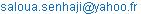(S. Senhaji)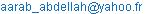(A. Aarab)

To cite this article:

Saloua Senhaji, Abdellah Aarab. A New Edge Detection using Decomposition Model. International Journal of Intelligent Information Systems. Special Issue: Academic Research for Multidisciplinary. Vol. 5, No. 3-1, 2016, pp. 28-31. doi: 10.11648/j.ijiis.s.2016050301.14

Received: December 19, 2015, 2016; Accepted: December 25, 2015; Published: June 30, 2016

Abstract: Edge detection is one of the most commonly used operations in image analysis, and there are more algorithms in the literature for enhancing and detecting edges. Natural images contain both textured and untextured regions, so the cues of contour and texture are exploited simultaneously. In this paper, we present a new edge detection method for natural images using decomposition model. The main idea is to decompose image in to two image components (geometric and texture) obtained by the PDE. The edge detection is performed not on the original image but on its geometric components. Experimental results on a wide range of images are shown.

Keywords: Partial Differential Equations, EDP, Decomposition Model, Geometrical Component, Edge Detector

Contents

1. Introduction

Human vision seems to make use of many sources of information to detect and recognize an object in an image. Researchers agree that edge and region information are utilized to extract a "perceptual unit" in image . Classical methods of edge detection [3,4,5] involve convolving the image with a filter (Sobel, Prewitt, Roberts,..), which is constructed to be sensitive to large gradients in the image while returning values of zero in uniform regions. There is an extremely large number of edge detection operators [2,6,7] available, each designed to be sensitive to certain types of edges. Variables involved in the selection of an edge detection operator include Edge structure, Noise environment and Edge orientation. The geometry of the operator determines a characteristic direction in which it is most sensitive to edges. Operators can be optimized to look for different direction edges. Edge detection is difficult in texture images, since both the texture and the edges contain high frequency content. To overcome this problem we propose to use the Aujol decomposition model. This decomposition is based on the mathematical model in the PDE introduced by Meyer  which split an image into a geometrical and texture components. The basic idea of our method is to use only the geometric component for edge detection. This method has given us better results compared to the same detector applied to the original image.

This paper is organized as follows: Section II presents edge detection techniques, Section III explains the decomposition models of an image, Section IV presents Experimental results, and finally Section V reports the conclusions.

2. Edge Detection Techniques

2.1. Roberts Edge Detection

The Roberts edge detection is introduced by Lawrence Roberts in 1965. This filter is the simplest. It performs a simple, quick to compute, 2-D spatial gradient measurement on an image. This method emphasizes regions of high spatial frequency which often correspond to edges. The input to the operator is a gray scale image the same as to the output is the most common usage for this technique. Pixel values in every point in the output represent the estimated complete magnitude of the spatial gradient of the input image at that point. This corresponds to the following convolution kernel:2.2. Sobel Edge Detection

The Sobel edge detection method was introduced by Sobel in 1970 

The Sobel operator performs a 2-D spatial gradient measurement on an image and so emphasizes regions of high spatial frequency that correspond to edges. Typically it is used to find the approximate absolute gradient magnitude at each point in an input gray scale image.. In conjecture at least, the operator consists of a pair of 3×3 convolution kernels. One kernel is simply the other rotated by 90°. This is very alike to the Roberts operator.The main interest of these masks is their ease of implementation and speed of processing. Their disadvantage is their high sensitivity to noise like the previous one.

2.3. Prewitt Edge Detection

The Prewitt edge detection was proposed by Prewitt in 1970. The Prewitt kernels are based on the idea of the central difference: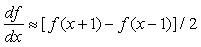Or for two-dimensional images: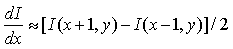This corresponds to the following convolution kernel: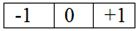These kernels are, however, sensitive to noise. we can reduce some of the effects of noise by averaging.. This is done in the Prewitt kernels by averaging in y when calculating and by averaging in x when calculating these produce the following kernels: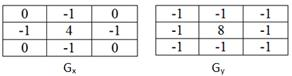3. Decomposition Model

Let u be the true image and f the distorted image. Then the model of ROF invented by Rudin, Osher and Fatemito solve is: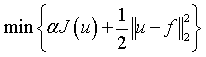Where J is the Total Variation regularization: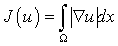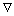denotes the spatial gradient.α measures the tradeoff between the regularization and the best fit to the noisy data.

This model decompose an image f into a component u and a component v= f - u; which is supposed to be the noise. In  Meyer out some limitations of the ROF model.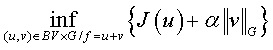Where BV is the space of functions of bounded variation  and G is the space of oscillating functions (in particular textures and noise).

Inspired by these works, many numerical algorithms have been developed to carry out the decomposition of gray scale images ,  and .

In this article, we adopt the algorithm proposed by Aujol and al.  to solve Meyer's model. This latter is numerically stable and mathematically convergent. It is constructed by minimizing a convex functional which depends on the two variables u and v, alternately in each variable. Each minimization is based on a projection algorithm to minimize the total variation.

Thus decomposition is given by minimizing a functional F:u BV containing the structure of the image, a second one, v G the texture.

Where J(u) is the total variation related to the extraction of the geometrical component,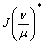is the Legendre-Fenche transform of J .

The parameter λ controls the L2−norm of the residual f - u - v. He smaller λ is, the smaller the L2 norm of the residual f - u - v is. The larger µ is, the more v contains information, and therefore the more u is averaged. Figure 1 shows the results of this algorithm with λ = 0,5 and µ = 140.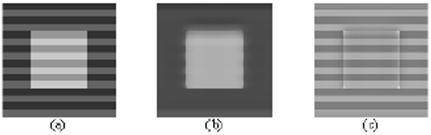Figure 1. (a) original image, (b) geometrical component, (c) texture component.

4. Experimental Result

In this section, we present our approach to edge detection, to show its effectiveness, we apply three image types, the first type has an image that contains only the texture, the second type has an image that contains texture and the object, the third type presents a noisy textured image. Figures 2 and 3 show these images with these components (geometric and texture) obtained by the decomposition model. The traditional edges detection operators (Sobel operator, Prewitt operator, Robert operator) results for each type of image and our method that use only the geometrical component to edge detection for the same operators are shown in figures 4 and 5.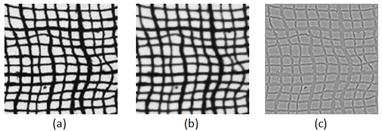Figure 2. (a) original image, (b) geometrical component, (c) texture component.Figure 3. (a) original image, (b) geometrical component, (c) texture component.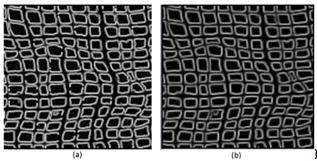Figure 4. (a) Robert edge detection using original image, (b) Robert edge detection using geometrical component.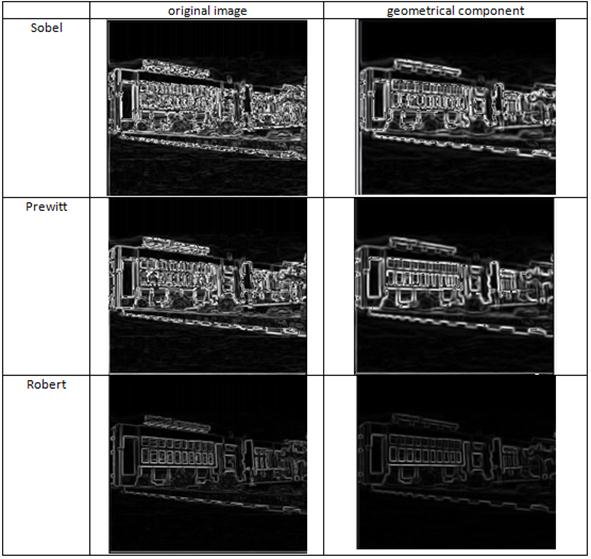Figure 5. Results of edge detection methods for textured imageusing original imageand geometrical component.

5. Conclusion

Although point and line detection certainly are important in any discussion on segmentation, edge detection is by far the most common approach for detecting meaningful discontinuities in gray level.

In this paper, we have presented an edge detection method using decomposition of the images. It consists in splitting an image into two components where the first contains the texture and the second is referred to geometrical characteristic. We use only the geometric component for edge detection. This method has given us better results compared to the same detector applied to the original image.

References

1. D. Mumford, S. M. Kosslyn, L. A. Hillger, and R. J. Hernstein, "Discriminating figure from ground: The role of edge detection and region growing," in Proc. Nat. Acad. Sci. USA, vol. 84, 1987, pp. 7354–7358.
2. Canny, J. A computational approach to edge detection. IEEE Trans. Pat. Anal. Mach. Intell., 8(6):679–698,1986.
3. N. Ahuja. A transform for multiscale image segmentation by integrated edge and region detection. IEEE TPAMI,18(12):1211–1235, 1996.
4. L. Wolf, X. Huang, I. Martin, and D. Metaxas. Patch-based texture edges and segmentation. In ECCV, pages II: 481–493, 2006. 3.
5. J. Matthews. "An introduction to edge detection: The sobel edge detector," Available at http://www.generation5.org/content/2002/im01.asp, 2002.
6. M.C. Shin, D. Goldgof, and K.W. Bowyer."Comparison of Edge Detector Performance through Use in an Object Recognition Task". Computer Vision and Image Understanding, vol. 84, no. 1, pp. 160-178, Oct. 2001.
7. R, Raskar; Tan, K-H; Feris, R.; Yu, J.; Turk, M., "Non-photorealistic Camera:Depth Edge Detection and Stylized Rendering Using Multi-Flash Imaging", ACM SIGGRAPH, August 2004
8. Y. Meyer. OscillatingPatternsinImageProcessingandNonlinear Evolution Equations.The Fifteenth Dean Jacqueline B. Lewis Memorial Lectures. Vol. 22 of University Lecture Series, AMS, Providence, 2001.
9. A. Chambolle. An algorithm for Total Variation Minimization and application. Journal of Mathematical Imaging and vision 20:89-97, 2004.
10. J.F. Aujol, G. Aubert, L. Blanc-Féraud, and A. Chambolle. Decomposing an image: Application to textured images and SAR images. Rapport technique, Université de Nice Sophia-Antipolis, 2003.
11. L. A. Vese and S. J. Osher. Modeling textures with total variation minimization and oscillating patterns in image processing. Journal of Scientific Computing, 19(1-3), 2003, pp. 553-572.
12. S.J. Osher, A. Sole, and L. A. Vese,Image decomposition and restoration using total variation minimization and the H(-1) norm.multiscalemodelling and simulation, ASIAM interdisciplinary journal, 1(3): 349-370, 2003.
13. E. Sobel, Camera Models and Machine Perception, PhD thesis, Stanford Univ., 1970.

 Contents 1. 2. 2.1. 2.2. 2.3. 3. 4. 5.
Article ToolsAbstractPDF(1778K)
Follow on us
PUBLICATION SERVICE
JOIN US
RESOURCES
SPECIAL SERVICES
ADDRESS
Science Publishing Group
548 FASHION AVENUE
NEW YORK, NY 10018
U.S.A.
Tel: (001)347-688-8931